# 【深度学习基础】第四十课：循环神经网络

## 循环神经网络

Posted by x-jeff on November 22, 2020

【深度学习基础】系列博客为学习Coursera上吴恩达深度学习课程所做的课程笔记。

# 1.循环神经网络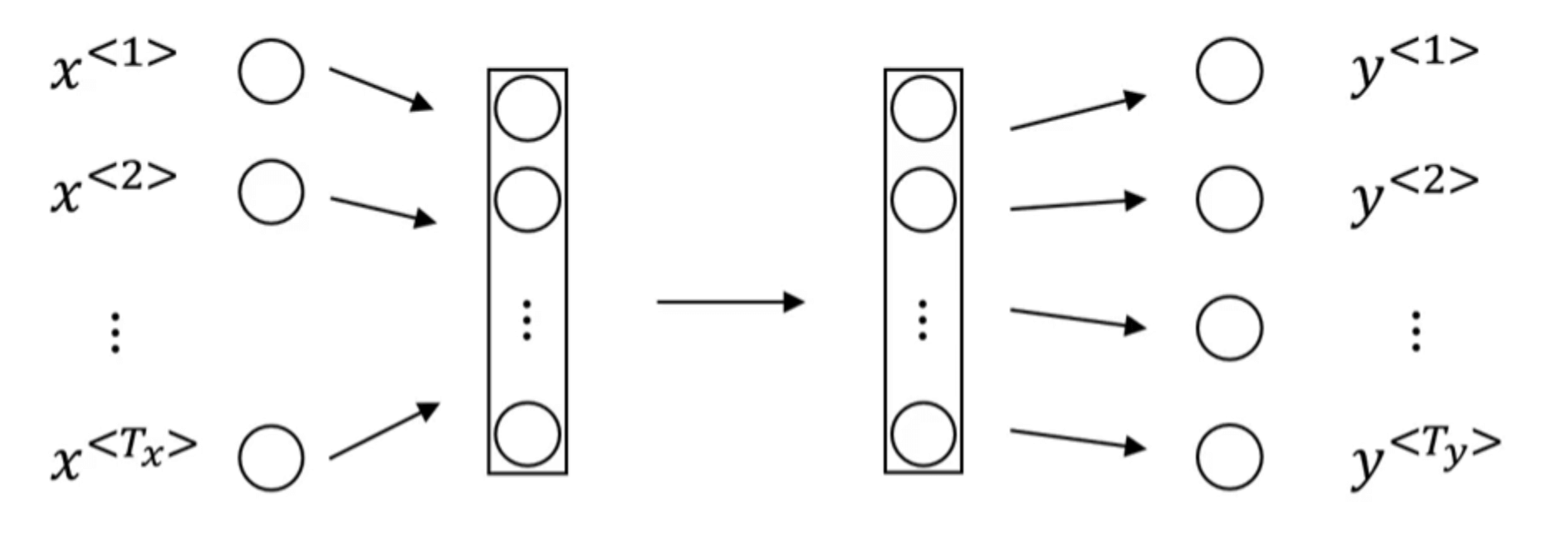1. 在不同的样本中，输入$x$和输出$y$可能有不同的长度。即使我们规定了$x,y$的最大长度，对于不满足最大长度的样本进行填充（pad），例如用0填充，使其满足最大长度，但这样依然不是一个好的解决办法。
2. 这种传统的标准神经网络结构并不能共享特征之间的信息，即忽视了序列信息，例如句子中前后单词之间的关联。并且模型的参数也会较多。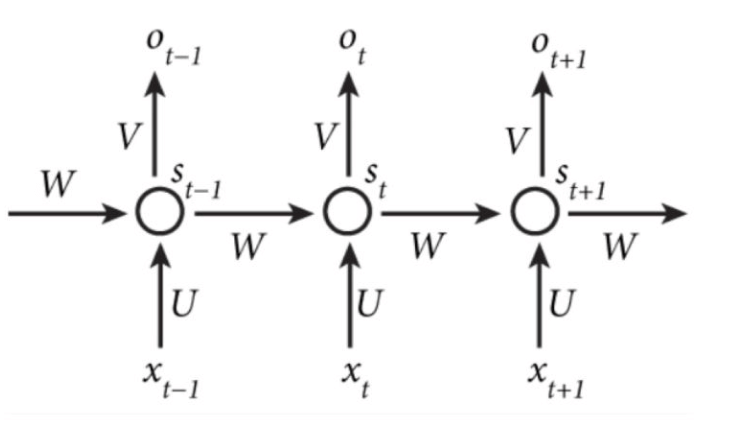• $O_t=g(V \cdot S_t+b_O)$
• $S_t=f(U \cdot X_t + W \cdot S_{t-1}+b_S)$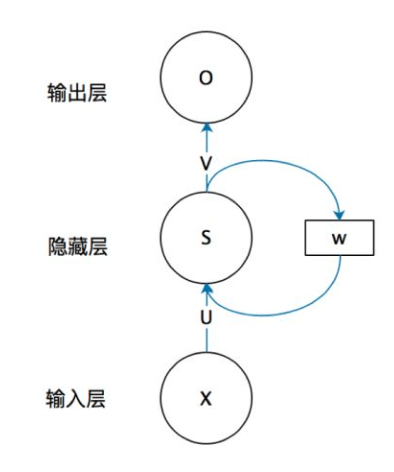$f$通常为tanh函数（偶尔也用ReLU函数）。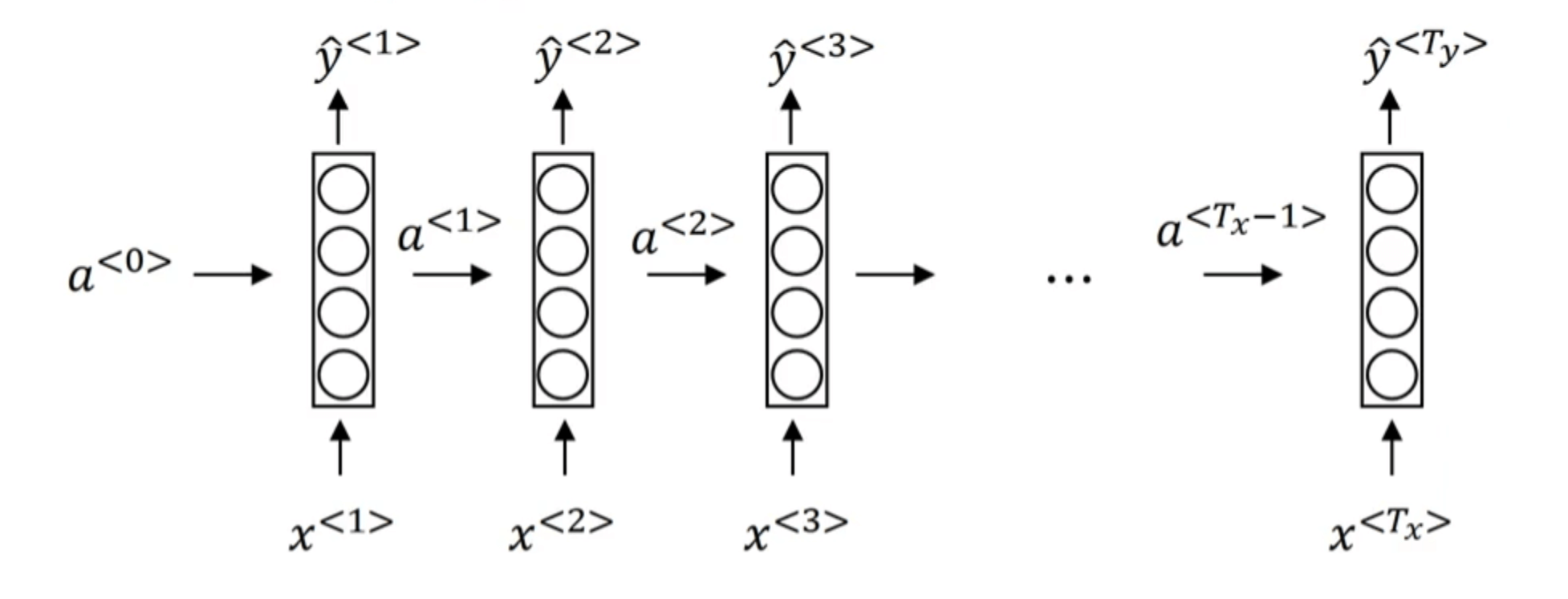$a^{<0>}$通常被初始化为零向量（或者随机初始化也可以）。比如在预测$\hat{y} ^{<3>}$时，模型会用到$x^{<1>},x^{<2>},x^{<3>}$的信息。

loss function可定义为交叉熵损失函数

$L^{<t>}(\hat {y} ^{<t>},y^{<t>})=-y^{<t>} \log \hat {y} ^{<t>} - (1-y^{<t>}) \log (1-\hat {y} ^{<t>})$ $L(\hat {y},y)=\sum_{t=1}^{T_x} L^{<t>}(\hat {y} ^{<t>},y^{<t>})$

# 2.更多的RNN框架

1. Many-to-One：比如情感分类问题。输入为一段文本，输出为评价等级（例如1星到5星）。
2. One-to-Many：比如音乐生成。输入为一种音乐风格，输出为一段音乐。
3. Many-to-Many，$T_x \neq T_y$：比如机器翻译。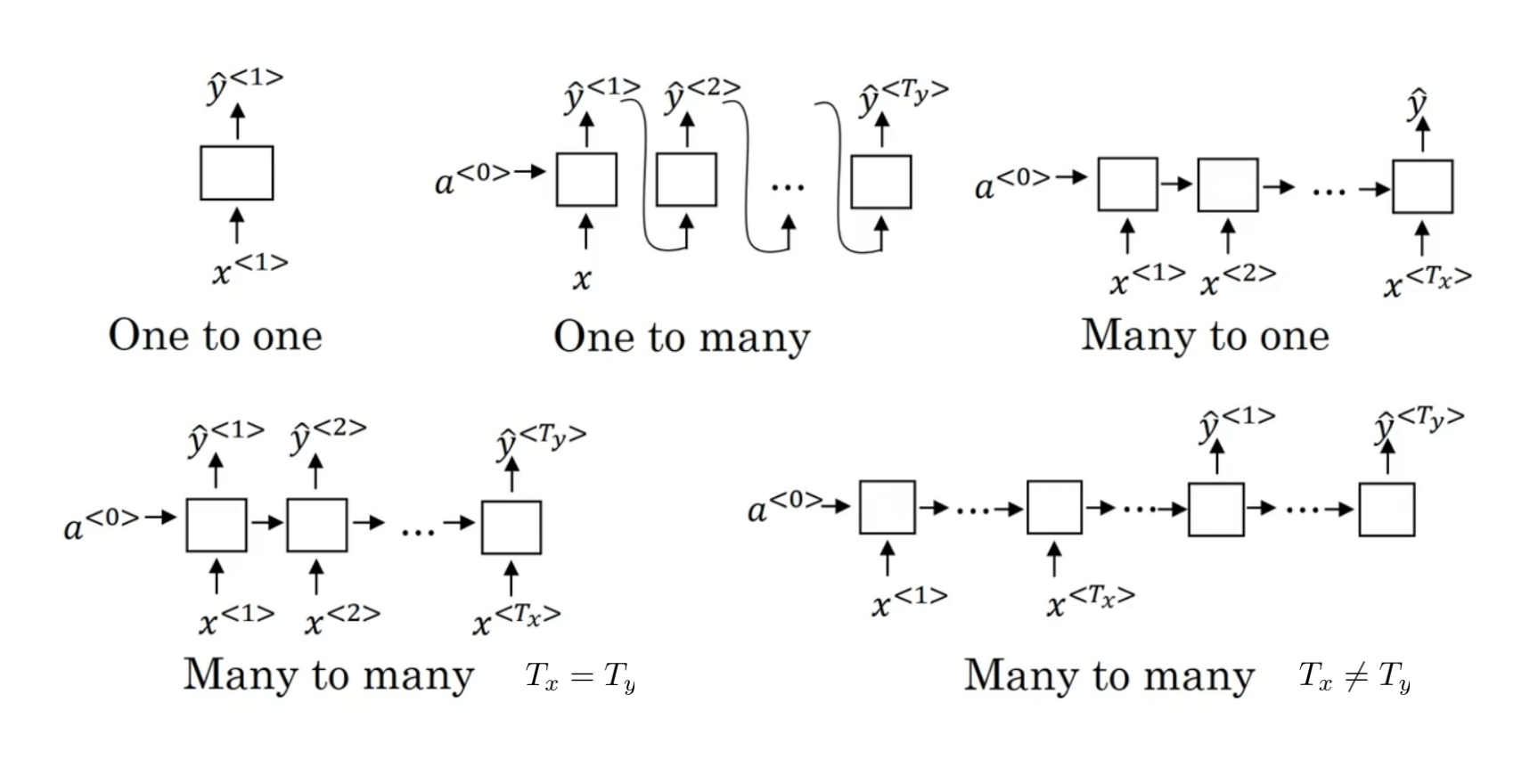# 3.参考资料